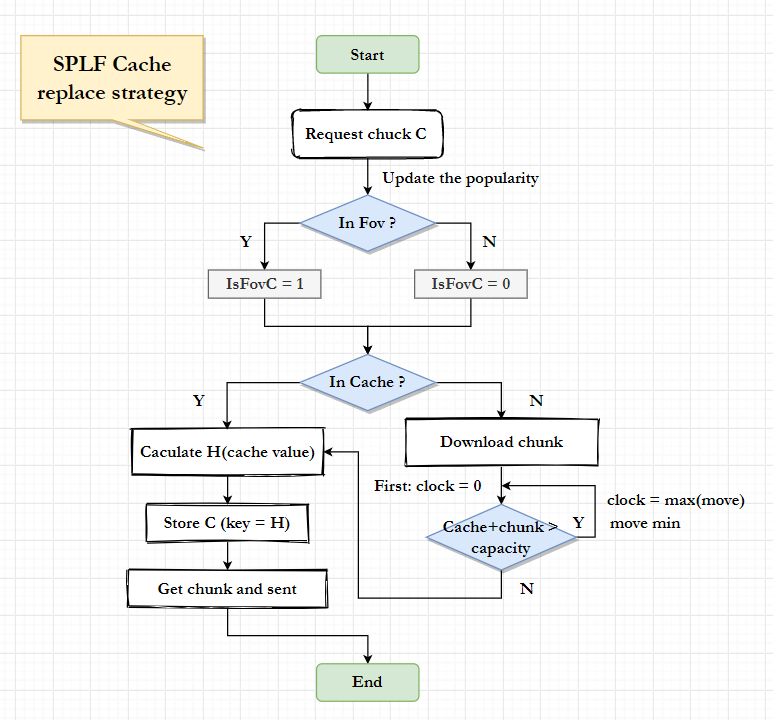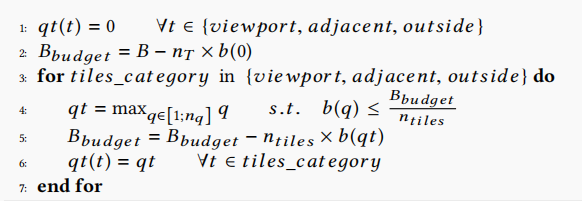Part One：A strategy for edge replacement

1、论文介绍

Requerement:

• ultra-high bandwidth
• ultra-low Motion-to-Photon (MTP)delay (减少头部运动和视频渲染的延迟) 需要 MTP <= 20ms，防止生理晕眩。

Problems to solve :

• 如何在有限缓存大小的边缘服务器的本地缓存中选择合适的视频表示
• 当缓存不足时应该替换哪个质量的块表示

1. 因为低层视频块的请求量较大，当超过缓存容量时，低层视频块被驱逐的概率要比高层视频块小。
2. 如果数据块在用户的FoV中，它将具有较低的驱逐优先级。
3. 受欢迎度较高的块应该以较高的概率缓存
4. 研究发现，替换一个大的内容块比替换多个小的内容块更能提高命中率。（考虑视频块大小）
5. An aging factor

2、系统架构

System Architecture

(1) 网络管理方案

• cache hit
• cache miss

Our goal: increase the cache hit

(2) 360°视频模型

• 视频信息Video information
• Viewpoint：来着用户以前的视角
• Network throughput: 网络吞吐量，从之前下载的块中测量
• Buffer status：以秒为单位

Output：（调度策略）

• 视角
• 质量调整

3、替换策略和方法

(1) 计算视频块的Cache Value

Cache Value：缓存这个视频的可能性。越小越应该被替换掉。

$$H\left(t, C_{v, k, i, q}\right)= C_{v, k, i, q}段视频在时间t的Cache Value$$

\begin{aligned} H\left(t, C_{v, k, i, q}\right)=& \text { clock }+P_{C_{v, k, i, q}}(t) \times \frac{1}{\operatorname{size}_{C_{v, k, i, q}}} \times \frac{L-q}{L} \times \frac{1+i s f o v_{C_{v, k, i, q}}}{2} \end{aligned}

$$P_{C_{v, k, i, q}}(t) 表示C_{v, k, i, q}段视频在时间t的流行程度$$

$$P_{C_{v, k, i, q}}(t)=\left\{\begin{array}{ll} 1, & \text { if } n=1 \\ 1+\sum_{j=1}^{n-1} \frac{1}{t-t_{j}}, & \text { if } n>1 \end{array}\right.$$

$${size}_{C_{v, k, i, q}}表示此块视频的大小$$

$$L是等级数量，q是当前视频的等级。$$

$$i s f o v_{C_{v, k, i, q}}是一个表示是否在预测Fov内的bool值$$

$$Clock\ 是 \ cache\ aging\ factor$$

(2) SPLF (Size-Popularity-Layer-Fov)缓存替换策略Part Two: 补充H.265、Tile-quality selection

1、H.265 Video Tiling

Tile的方法比Non-tile的方法显著减少存储空间和带宽。2 polar tiles and 4 equatorial tiles。

$$S_{\text {tiled }}=d \times\left(b_{1}+b_{0}\right) \times \alpha\left(n_{t}\right)$$

$$\alpha\left(n_{t}\right)表示平铺过程引入的编码开销,d是视频时长，level(0 \to n_{q})$$

\begin{aligned} S_{\text {non-tiled }} &=d \times\left[\frac{w_{p} \times h_{p}}{w \times h} \times b_{1}+\left(1-\frac{w_{p} \times h_{p}}{w \times h}\right) \times b_{0}\right] \times N\left(w, h, w_{p}, h_{p}, \xi\right) \end{aligned}

$$w_{p}和h_{p}是视角的宽和高，w和h是整个视频的宽和高，\\ N\left(w, h, w_{p}, h_{p}, \xi\right)是不同视角需要编码的数量$$

\begin{aligned} G=\frac{S_{\text {non-tiled }}}{S_{\text {tiled }}} &=\frac{b_{1}-b_{0}}{b_{1}+b_{0}} \times \frac{w_{p} \times h_{p}}{w \times h} \times \frac{N\left(w, h, w_{p}, h_{p}, \xi\right)}{\alpha\left(n_{t}\right)}+\\ &+\frac{b_{0}}{b_{0}+b_{1}} \times \frac{N\left(w, h, w_{p}, h_{p}, \xi\right)}{\alpha\left(n_{t}\right)} \end{aligned}

2、Tiles Quality Selection

$$\begin{array}{c} p(k+\Delta)=p(k)+\Delta \times \widehat{p(k)} \\ \widehat{p(k)}=\frac{p(k)-p(k-\delta)}{\delta} \end{array}$$

$$\Delta是视频片段的持续时间，\delta是测量速度的时间间隔(100ms)，\widehat{p(k)}是速度$$Last modification：March 7th, 2021 at 08:38 pm